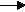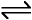gcsescience.com                                          22                                          gcsescience.com

Acids and Alkalis

Examples of Alkalis.

The three common alkalis you will find in the laboratory are

1) Sodium hydroxide solution - NaOH(aq).

2) Calcium hydroxide solution - Ca(OH)2(aq) is called lime water
(lime water is used in the test for carbon dioxide gas).

3) Ammonia solution - NH3(aq).

1 and 2 are strong alkalis, 3 is a weak alkali - see pH.
They all ionise in water to form hydroxide ions (OH- ions).

1) NaOH(aq)Na+(aq)    +      OH-(aq)

2) Ca(OH)2(aq)Ca2+(aq)    +     2OH-(aq)

3) NH3(aq)  +   H2O(l)NH4+(aq)   +   OH-(aq)
NH4+ is called an ammonium ion.

Any metal oxide or hydroxide is a base.
If the base dissolves in water it is called an alkali
(compare this with non-metal oxides).

gcsescience.com       The Periodic Table       Index       Alkali Quiz       gcsescience.com